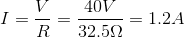# AP Physics C: Mechanics : Using Ohm's Law

## Example Questions

### Example Question #1 : Circuit Relationships

A cicuit with a voltage source of 40V has a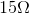resistor and a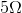resistor in series, followed by tworesistors in parallel. After the parallel branches rejoin, there is a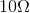resistor before the circuit is closed at the voltage source.

What is the current supplied by the source?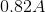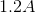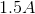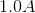Explanation:

The current supplied by the source can be calculated using a derivation of Ohm's law: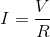Start by finding the equivalent resistance of the circuit.

Sum the first two resistors in series: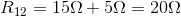Calculate the equivalent resistance of the two resistors in parallel: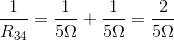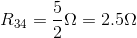Now, all of the reistances can be viewed as being in series.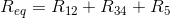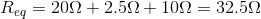Returning to our current calulation, we can find our final answer: